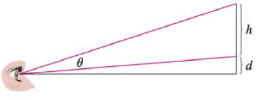Chapter 4.7, Problem 78E### Single Variable Calculus: Early Tr...

8th Edition
James Stewart
ISBN: 9781305270343

#### Solutions

Chapter
Section### Single Variable Calculus: Early Tr...

8th Edition
James Stewart
ISBN: 9781305270343
Textbook Problem

# A painting in an art gallery has height h and is hung so that its lower edge is a distance d above the eye of an observer (as in the figure). How far from the wall should the observer stand to get the best view? (In other words, where should the observer stand so as to maximize the angle θ subtended at his eye by the painting?)To determine

To find: The distance from the observer to the painting.

Explanation

Given:

The height of the painting =h

The distance between the lower edge of the painting and the eye of the observer = d.

Calculation:

Consider the triangle ABC

CAB=α

tanα=dx

Consider the triangle ACD

In Figure 1

In triangle ABD,

Then,

tan(θ+α)=h+dx

So,

tan(θ+α)=tanθ+tanα1tanθtanαh+dx=dx+tanθ1dxtanθ(hx+dx)(1dxtanθ)=dx+tanθhxhdx2tanθ+dxd2x2tanθ=dx+tanθ

Simplify that,

hdx2tanθd2x2tanθtanθ=hxtanθ(hdx2+d2x2+1)=hxtanθ(hd+d2+x2x2)=hxtanθ(hd+d2+x2x)=h

Therefore,

tanθ(x+hdx+d2x)=htanθ=h(x+hdx+d2x)=hx2+hd+d2x=hxx2+hd+d2

Differentiate with respect to θ ,

sec2θdθdx=(x2+hd+d2)hhx(2x)(x2+hd+d2)2

For critical points, dθdx=0

(x2+hd+d2)hhx(2x)(x2+hd+d2)2=0hx2+h2d+d2h2hx2=0h2d+d2h=hx2

Simplify that,

h2d+d2h=hx2x2=hd+d2x=hd+d2tanθ=hxx2+hd+d2

Squaring both sides,

tan2θ=h2x2(x2+hd+d2)21+tan2θ=1+h2x2(x2+hd+d2)2sec2θ=(x2+hd+d2)2+h2x2(x2+hd+d2)2=x4+h2d2+d4+h2x2(x2+hd+d2)2

Substitute sec2θ=x4+h2d2+d4+h2x2(x2+

### Still sussing out bartleby?

Check out a sample textbook solution.

See a sample solution

#### The Solution to Your Study Problems

Bartleby provides explanations to thousands of textbook problems written by our experts, many with advanced degrees!

Get Started

#### What are the two requirements for a random sample?

Statistics for The Behavioral Sciences (MindTap Course List)

#### ∫x−4dx = −4x−5 + C

Study Guide for Stewart's Single Variable Calculus: Early Transcendentals, 8th

#### For

Study Guide for Stewart's Multivariable Calculus, 8th